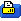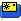Dec 08, 2019, 02:14 PM
Registered User
Quote:
 Originally Posted by momo5400 Hi David, the graph at the beginning of the video is the speed of the Canmore GT760FL gps datalogger, not good ???? I think I'm going to build this with SD card with arduino nano and RTC module. what do you think of this one? https://create.arduino.cc/projecthub...81527&offset=1 regards Yves
Hi Yves,
I assumed the data was from the Canmore but I believe it is also only storing location and time (like the Google Earth .gpx file) so to be accurate it needs a good displacement between sample periods. At 1 second sampling it should be fairly accurate but I wouldn't trust any results faster than this.
The project looks good but I'm not too familiar with Arduino or how to find the include files they indicate. It looks like any GPS with 9600 baud and NMEA would work so the BN-180 or BN-220 would be fine and save a bit of weight and space. I included a low speed cut off value in my code so the device wouldn't record to EEPROM until the speed was over 5km/h. I must check out that display - I've been looking for a small display.

Cheers,
David
 Sign up now to remove ads between postsDec 08, 2019, 03:20 PM Registered User OK I found the include files and can sort of follow it. It appears they are parsing two sentences - RMC and GGA. The RMC sentence contains the field for speed however the value returned is in knots and I can't see where they convert this km/h. It needs the knots multiplied by 1.852 (floating point) or 463/250 (integer maths). Perhaps someone can point out to me where this takes place. Cheers, David
Dec 09, 2019, 11:05 AM
Registered User
Quote:
 Originally Posted by KiwiDavid OK I found the include files and can sort of follow it. It appears they are parsing two sentences - RMC and GGA. The RMC sentence contains the field for speed however the value returned is in knots and I can't see where they convert this km/h. It needs the knots multiplied by 1.852 (floating point) or 463/250 (integer maths). Perhaps someone can point out to me where this takes place. Cheers, David
Hi David,
this small screen is good and ultra low weight

in this code you have all the data read from the gps (neo-6m)
/*
* Rui Santos
* Complete Project Details http://randomnerdtutorials.com
*
* Based on the example TinyGPS++ from arduiniana.org
*
*/

#include <TinyGPS++.h>
#include <SoftwareSerial.h>

static const int RXPin = 4, TXPin = 3;
static const uint32_t GPSBaud = 9600;

// The TinyGPS++ object
TinyGPSPlus gps;

// The serial connection to the GPS device
SoftwareSerial ss(RXPin, TXPin);

void setup(){
Serial.begin(9600);
ss.begin(GPSBaud);
}

void loop(){
// This sketch displays information every time a new sentence is correctly encoded.
while (ss.available() > 0){
if (gps.location.isUpdated()){
// Latitude in degrees (double)
Serial.print("Latitude= ");
Serial.print(gps.location.lat(), 6);
// Longitude in degrees (double)
Serial.print(" Longitude= ");
Serial.println(gps.location.lng(), 6);

// Raw latitude in whole degrees
Serial.print("Raw latitude = ");
Serial.print(gps.location.rawLat().negative ? "-" : "+");
Serial.println(gps.location.rawLat().deg);
// ... and billionths (u16/u32)
Serial.println(gps.location.rawLat().billionths);

// Raw longitude in whole degrees
Serial.print("Raw longitude = ");
Serial.print(gps.location.rawLng().negative ? "-" : "+");
Serial.println(gps.location.rawLng().deg);
// ... and billionths (u16/u32)
Serial.println(gps.location.rawLng().billionths);

// Raw date in DDMMYY format (u32)
Serial.print("Raw date DDMMYY = ");
Serial.println(gps.date.value());

// Year (2000+) (u16)
Serial.print("Year = ");
Serial.println(gps.date.year());
// Month (1-12) (u8)
Serial.print("Month = ");
Serial.println(gps.date.month());
// Day (1-31) (u8)
Serial.print("Day = ");
Serial.println(gps.date.day());

// Raw time in HHMMSSCC format (u32)
Serial.print("Raw time in HHMMSSCC = ");
Serial.println(gps.time.value());

// Hour (0-23) (u8)
Serial.print("Hour = ");
Serial.println(gps.time.hour());
// Minute (0-59) (u8)
Serial.print("Minute = ");
Serial.println(gps.time.minute());
// Second (0-59) (u8)
Serial.print("Second = ");
Serial.println(gps.time.second());
// 100ths of a second (0-99) (u8)
Serial.print("Centisecond = ");
Serial.println(gps.time.centisecond());

// Raw speed in 100ths of a knot (i32)
Serial.print("Raw speed in 100ths/knot = ");
Serial.println(gps.speed.value());
// Speed in knots (double)
Serial.print("Speed in knots/h = ");
Serial.println(gps.speed.knots());
// Speed in miles per hour (double)
Serial.print("Speed in miles/h = ");
Serial.println(gps.speed.mph());
// Speed in meters per second (double)
Serial.print("Speed in m/s = ");
Serial.println(gps.speed.mps());
// Speed in kilometers per hour (double)
Serial.print("Speed in km/h = ");
Serial.println(gps.speed.kmph());

// Raw course in 100ths of a degree (i32)
Serial.print("Raw course in degrees = ");
Serial.println(gps.course.value());
// Course in degrees (double)
Serial.print("Course in degrees = ");
Serial.println(gps.course.deg());

// Raw altitude in centimeters (i32)
Serial.print("Raw altitude in centimeters = ");
Serial.println(gps.altitude.value());
// Altitude in meters (double)
Serial.print("Altitude in meters = ");
Serial.println(gps.altitude.meters());
// Altitude in miles (double)
Serial.print("Altitude in miles = ");
Serial.println(gps.altitude.miles());
// Altitude in kilometers (double)
Serial.print("Altitude in kilometers = ");
Serial.println(gps.altitude.kilometers());
// Altitude in feet (double)
Serial.print("Altitude in feet = ");
Serial.println(gps.altitude.feet());

// Number of satellites in use (u32)
Serial.print("Number os satellites in use = ");
Serial.println(gps.satellites.value());

// Horizontal Dim. of Precision (100ths-i32)
Serial.print("HDOP = ");
Serial.println(gps.hdop.value());
}
}
}

regards
 Dec 09, 2019, 02:42 PM Registered User Hi, Thanks for sending that through. The TinyGPS code I stumbled across didn't have the extensive speed conversions stuff in it. What you sent is obvious now. You could also integrate velocity with respect to time and derive the total distance traveled. This is probably more accurate than using the maths intensive Great Circle distance between two locations method which suffers problems when displacement is small (low speeds) just like the speed issue you have previously had. I will check out the display - could be ideal for RC applications. Best regards, David
Mar 15, 2020, 10:19 AM
Registered User
 SKIPPER XL 4S 2200 mAh heavy duty (3 min 27 sec)
May 06, 2020, 05:05 AM
Registered User

# with the wind it's rock'n roll

 SKIPPER XL 4S 2200 mAh HEAVY DUTY (2 min 18 sec)
Aug 01, 2021, 09:31 AM
Registered User
 Thread ToolsShow Printable VersionEmail this Page